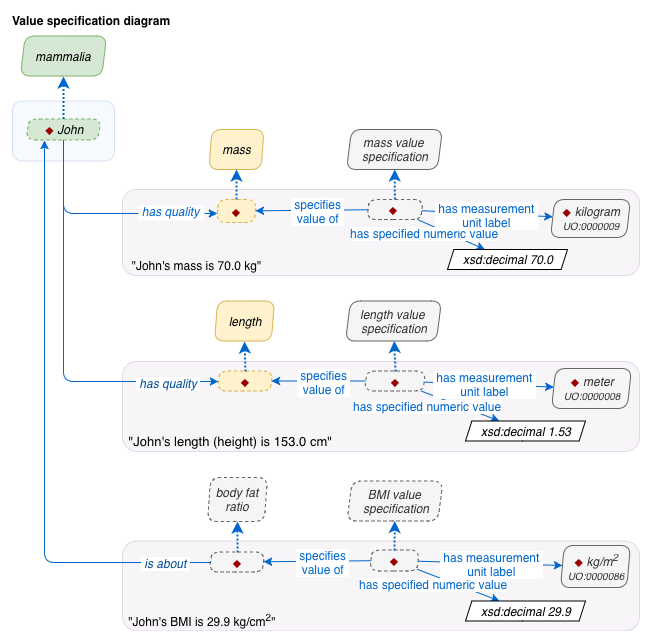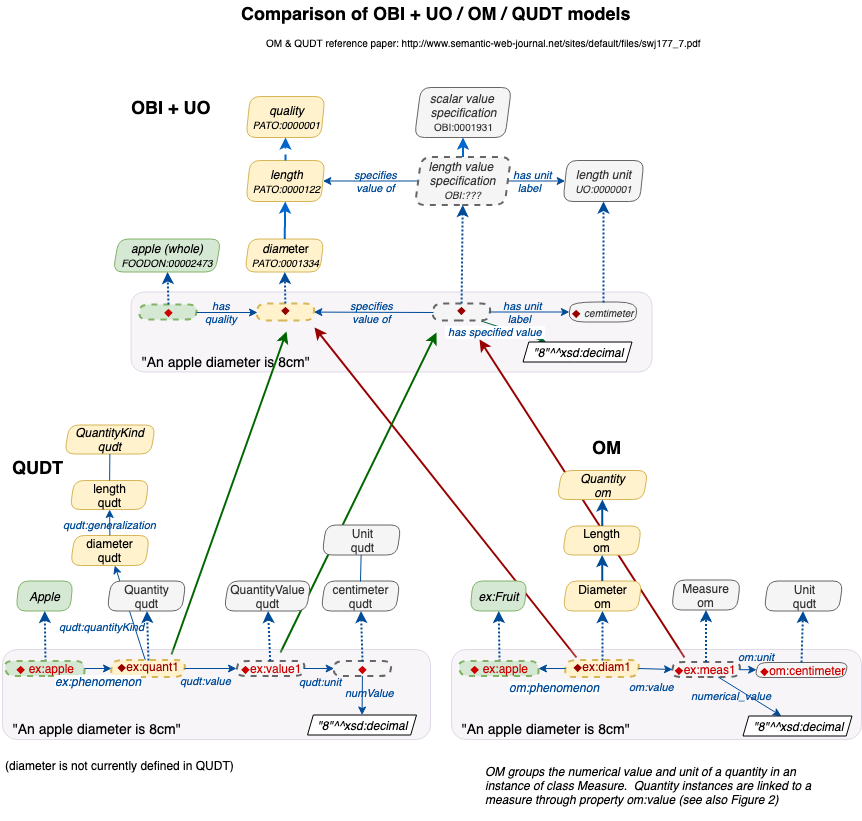# How to model quantities and measurements#

Measurements lie at the heart of science, as well as engineering and everyday life. However, there is no consensus data model or exchange standard for measurements. Even representation of units is challenging, with different competing standards.

There is unlikely to be a consensus data model, because different use cases and requirements drive different designs. Instead it may be fruitful to standardize on different composable portions of a data model, and to enumerate design patterns (and anti-patterns), with a clear understanding of pros and cons, and how to map between them.

Here we will walk through how to model measurements for a human research subjects, making decisions based on use cases. Although our central example is a human or organismal subject, the patterns should carry forward to other subjects, ranging from environmental sites through to physical parts of engineered systems.

Although this how-to guide is intended primarily for modeling using LinkML, the general patterns here are broadly applicable.

## Central Example#

The central example used here is measurements of a few properties that can be recorded for people, in particular:

• body mass/weight

• height

• BMI (body-mass index)

But these should be generalizable to other properties or other kinds of observations

## Requirements#

There are multiple ways to make a data model or standard that uses measurements. The decision should be made based on requirements, use cases, and the overall context of how the data model should be used.

For our purposes here, the following features/requirements can help us:

1. Does the data need to be computed over?

2. Does the data need to be exchanged in a flat table or array form, or can it be normalized?

3. Do the scalar values need to be immediately accessible?

4. Is it necessary to represent error bars or ranges?

5. Does each individual measurement instance need to have metadata associated with it?

6. Are the units fixed for each measurement type within the scope of the standard, or can different units be used?

## Core schema#

We will work through a number of different alternatives, but all use the following core skeleton:

```id: https://w3id.org/linkml/howtos/measurements
name: research_subject_measurements
title: A demonstrator schema for measuring properties of research subjects

prefixes:
sh: https://w3id.org/shacl/
UO: http://purl.obolibrary.org/obo/UO_
PATO: http://purl.obolibrary.org/obo/PATO_
qudt: http://qudt.org/schema/qudt/

default_prefix: ex
default_range: string

classes:
Subject:
description: A research subject
attributes:
id:
identifier: true
description: A unique identifier for the research subject
```

## Patterns and Anti-patterns#

Next we’ll take a look at different ways to model a simple hypothetical standard, where we have research subjects (e.g. people, or potentially experimental organsisms) with a number of measurements. For the basic use case we don’t assume time-series data.

For each approach, we show a schema snippet, and example data in both YAML/JSON and in tabular form.

### Simple Explicit Scalar Pattern#

The simplest possible model has each measurement modeled as a single scalar value:

```classes:
Subject:
attributes:
id:
identifier: true
mass_in_kg:
description: the whole-body mass, measured at one point during the study
slot_uri: OBA:VT0001259
range: decimal
unit:
ucum_code: kg
has_quantity_kind: PATO:0000125 ## mass
height_in_m:
description: the height of the subject
slot_uri: OBA:VT0001253
range: decimal
unit:
ucum_code: m
has_quantity_kind: PATO:0000119 ## height
bmi:
range: decimal
unit:
ucum_code: kg/m2
has_quantity_kind: NCIT:C16358
```

Some aspects of the above schema are optional but provide useful metadata, e.g

• we annotate each unit using unit

• we annotate each slot with slot_uri

These don’t modify the overall structure of the data model, so we will focus less on these from here on

Example data in YAML/JSON:

```- id: P001
mass_in_kg: 70.0
height_in_m: 1.53
bmi: 29.9
```

Tabular:

id

mass_in_kg

height_in_m

bmi

P001

70.0

1.53

29.9

Properties:

• nesting levels: 0

• fixed_units: y

• ranges: n

• computable scalars: y

• safe: y

Here “nesting levels” of zero means that the data is flat and is directly serializable in a tabular form without additional mapping or transformation.

Units are fixed in that the schema dictates mass must be in kg. If you have mass in lbs, then it must be converted prior to populating data objects conformat to the model

Ranges are not supported in this data model (we will look at ways to extend this below)

### Variant: Simple Implicit Scalar Pattern#

This is a variant of the above pattern, except the unit name is not baked into the field name:

```classes:
Subject:
attributes:
id:
identifier: true
mass:
range: decimal
unit:
ucum_code: kg
height:
range: decimal
unit:
ucum_code: m
bmi:
range: decimal
unit:
ucum_code: kg/m2
```

Example data in YAML/JSON:

```- id: P001
mass: 70.0
height: 1.53
bmi: 29.9
```

Tabular:

id

mass

height

bmi

P001

70.0

1.53

29.9

This is structurally equivalent to the previous data model - all we have done is make the slot names more concise. However, this makes this an anti-pattern

Why this is an anti-pattern:

Even though the unit is explicit in the schema, if the data becomes decoupled from the schema, then there is a risk of mis-interpretation. For this reason we mark this as unsafe in the model properties below.

Properties:

• nesting levels: 0

• fixed_units: y

• ranges: n

• computable scalars: y

• safe: n

### Simple Coupled Scalar Pattern#

For this example, consider our requirements have changed, and we want to record a range of body weights, for example the body weight at the start of the study, and at the end.

We can also imagine using an analogous structure to record ranges encompassing uncertainty. Or sometimes ranges might be a proxy for two things being measured. For example, a soil sample taken from the earth may have a range indicating the top and bottom of the column.

```classes:
Subject:
attributes:
id:
identifier: true
min_mass_in_kg:
group: mass
range: decimal
unit:
ucum_code: kg
has_quantity_kind: PATO:0000125 ## mass
max_mass_in_kg:
group: mass
range: decimal
unit:
ucum_code: kg
has_quantity_kind: PATO:0000125 ## mass
height_in_m:
range: decimal
unit:
ucum_code: m
has_quantity_kind: PATO:0000119 ## height
min_bmi:
group: bmi
range: decimal
unit:
ucum_code: kg/m2
has_quantity_kind: NCIT:C16358
max_bmi:
group: bmi
range: decimal
unit:
ucum_code: kg/m2
has_quantity_kind: NCIT:C16358
```

Example data in YAML/JSON:

```- id: P001
min_mass_in_kg: 70.0
max_in_kg: 72.0
height_in_m: 1.53
min_bmi: 29.9
max_bmi: 30.7
```

Tabular:

id

min_mass_in_kg

max_mass_in_kg

height_in_m

min_bmi

max_bmi

P001

70.0

72.0

1.53

29.9

30.7

Properties:

• nesting levels: 0

• fixed_units: y

• ranges: y

• computable scalars: y

• safe: y

• normal form: n

Like the previous example, this has zero levels of nesting, and can be trivially serialized as a table. The units are also fixed, but ranges are supported.

### Simple Flexible Unit Scalar Pattern#

Next we will look at a requirement to make units flexible, such that for example body weight can be specified ib kilograms or pounds.

```classes:
Subject:
attributes:
id:
identifier: true
mass:
range: decimal
mass_unit:
range: MassUnitEnum
height:
range: decimal
height_unit:
range: HeightUnitEnum
bmi:
range: decimal
unit:
ucum_code: kg/m2
```

Example data in YAML/JSON:

```- id: P001
mass: 70.0
mass_unit: kg
height: 1.53
height_unit: m
bmi: 29.9
```

Tabular:

id

mass

mass_unit

height

height_unit

bmi

P001

70.0

kg

1.53

m

29.9

Properties:

• nesting levels: 0

• fixed_units: n

• ranges: n

• computable scalars: y

• safe: partially

• normal form: n

### Flexible Unit Nested Measurement Pattern#

```classes:
Subject:
attributes:
id:
identifier: true
mass:
range: Measurement
height:
range: Measurement
bmi:
range: Measurement
Measurement:
attributes:
quantity_value:
range: decimal
quantity_unit:
```

Example data in YAML/JSON:

```- id: P001
mass:
quantity_value: 70.0
quantity_unit: kg
height:
quantity_value: 1.53
quantity_unit: m
bmi:
quantity_value: 29.9
quantity_unit: kg/m2
```

Properties:

• nesting levels: 1

• fixed_units: n

• ranges: n (but trivially modifiable to do this)

• computable scalars: y

• safe: y

• normal form: y

### Flexible Unit String Encoding Pattern#

```classes:
Subject:
attributes:
id:
identifier: true
mass:
range: string
structured_pattern:
syntax: "{float} {unit}"
height:
range: string
structured_pattern:
syntax: "{float} {unit}"
bmi:
range: string
structured_pattern:
syntax: "{float} {unit}"
```

Example data in YAML/JSON:

```- id: P001
mass: 70 kg
height: 1.53 m
bmi: 29.9 kg/m2
```

Tabular:

id

mass

height

bmi

P001

70.0 kg

1.53 m

29.9 kg/m2

Properties:

• nesting levels: 0

• fixed_units: n

• ranges: n (but somewhat modifiable to do this)

• computable scalars: N

• safe: y

• normal form: y

### Generic Quantity Type Pattern#

```classes:
Subject:
attributes:
id:
identifier: true
measurements:
range: Measurement
multivalued: true
Measurement:
attributes:
quantity_kind:
range: uriorcurie
quantity_value:
range: decimal
quantity_unit:

```

Example data in YAML/JSON:

```- id: P001
measurements:
- quantity_kind: body mass
quantity_value: 70
quantity_unit: kg
- quantity_kind:  height
quantity_value: 1.53
quantity_unit: m
- quantity_kind: bmi
quantity_value: 29.9
quantity_unit: kg/m2
```

Tabular:

narrow table

id

quantity_kind

quantity_value

quantity_unit

P001

body mass

70.0

kg

P001

height

1.53

m

P001

bmi

29.9

kg/m2

• nesting levels: 1

• fixed_units: n

• ranges: n (but trivially modifiable to do this)

• computable scalars: y

• safe: y

• normal form: y

• tabular serialization: narrow table

• open: y

## Case Studies#

### FHIR#

Fast Healthcare Interoperability Resources (FHIR) is a standard for Electronic Health Records (EHRs).

The FHIR Observation resource is intended for capturing measurements and subjective point-in-time assessments

Observations make use of the Quantity resource:

Example data instance:

```resourceType: Observation
id: example
text:
status: generated
status: final
category:
- coding:
- system: http://terminology.hl7.org/CodeSystem/observation-category
code: vital-signs
display: Vital Signs
code:
coding:
- system: http://loinc.org
code: 29463-7
display: Body Weight
- system: http://loinc.org
code: 3141-9
display: Body weight Measured
- system: http://snomed.info/sct
code: '27113001'
display: Body weight
- system: http://acme.org/devices/clinical-codes
code: body-weight
display: Body Weight
subject:
reference: Patient/example
encounter:
reference: Encounter/example
effectiveDateTime: '2016-03-28'
valueQuantity:
value: 185
unit: lbs
system: http://unitsofmeasure.org
code: "[lb_av]"
```

Each observation object nas a `valueQuantity` field which has both the value and the unit, together with a way of representing the unit standard (UCUM).

### MIxS#

The Genomics Standards Consortium (GSC) Minimal Information about any Sequence (MIxS) standard provides standardized metadata elements for recording properties of environmental and biomedical samples intended for sequencing. These properties are a mix of categorical values and quantities, for things that are measured.

MIxS provides terms such as:

• depth (e.g depth in the soil at which a sample was taken)

• altitude (height above sea level)

Historically MIxS has not sought to standardize on specific units (e.g. meters for depth). Instead each field is associated with a regular expression-style patterns.

Examples:

• depth: `{float} {unit}`

In contrast to FHIR which is an interoperability system for exchanging messages between machines, the choices in MIxS reflect the norms of interchange often involving simple spreadsheets.

Using MIxS users can have single fields for each property, allowing users to use a unit system of choice. However, the use of strings means values must be parsed.

### OBI#Comparison with QUDT and OM:data:

```- id: P001
type: NCBITaxon:9606
has_quality:
- type: PATO:0000125
has_measurement:
has measurement unit label: UO:0000009
has decimal value: 70.0
- type: PATO:0000122
has_measurement:
has measurement unit label: UO:0000008
has decimal value: 1.53
```

### OBOE#

Concepts:

• Observation: an event in which one or more measurements are taken

• Measurement: the measured value of a property for a specific object or phenomenon (e.g., 3.2)

• Entity: an object or phenomenon on which measurements are made (e.g., Quercus rubrum)

• Characteristic: the property being measured (e.g., VolumetricDensity)

• Standard: units and controlled vocabularies for interpreting measured values (e.g., g/cm^3)

• Protocol: the procedures followed to obtain measurements (e.g., DensityProtocol2014)